# Creating and visualizing spatial simulations of tumor growth using SITH

## Introduction and basic model

The cells within a cancerous tumor are usually highly diverse. An average tumor contains hundreds of thousands of mutations spread throughout billions of cancer cells, although it is thought that only a small percentage of these mutations are “drivers” which facilitate the progression of cancer into later stages (Greaves and Maley 2012). A lack of understanding about the evolutionary process which results in the observed intratumor heterogeneity is a major obstacle preventing the development of effective cancer therapies (Stanta and Bonin 2018).

Tumor growth is inherently a three-dimensional process. For this reason, the most accurate models of intratumor heterogeneity will factor in the effects that spatial growth has on the diversity of the tumor. One of the most popular models was introduced by Waclaw et. al. (2015), which models spatial tumor growth as a multi-type branching process where cells occupy sites on a 3D lattice. Similar models have been studied in more recent literature (See Chkhaidze et. al. (2019), Opasic et. al. (2018)). However, the software is either unavailable or designed to run at the command line, which is inconvenient for R users.

This package, a Spatial model of Intra-tumor Heterogeneity (SITH), implements a 3D lattice-based model of tumor growth (see Model details) that can be used entirely from the R console. The package allows for 3D interactive visualizations of the tumor, as well as a comprehensive summary of the spatial distribution of mutants within the tumor. SITH also contains functions allowing users to create simulated datasets from the generated tumor.

### Model details

Tumor growth is modeled as an exponential birth-death process where cells occupy sites on the three-dimensional integer lattice. Each cell is given a birth rate $$b$$ and a death rate $$d$$ such that the time until cell replication or death is exponentially distributed with parameters $$b$$ and $$d$$, respectively. A cell can replicate if at least one of the six sites adjacent to it is unoccupied. Each time cell replication occurs, both daughter cells acquire $$Pois(u)$$ mutations, for some chosen (usually small) $$u$$. We follow the “infinite alleles model” (Kimura and Crow 1964) in assuming that each alteration occurs only once, so that each mutation event induces a unique allele in the population.

An alteration is a driver mutation with probability $$du$$ and is a passenger otherwise. To model the selective advantage conferred to driver mutations, the birth rate of a cell depends on the number of driver mutations present in its genotype. Following a common assumption (Waclaw et. al. 2015), a cell with $$k$$ driver mutations is given a birth rate $$bs^k$$, where $$s \geq 1$$ and $$b$$ is the initial, or wild-type, birth rate. The death rate remains the same as the dynamics of the model are completely determined by $$b/d$$. Since cancerous tumors are believed to often begin with a single mutated cell (Cooper 2000), the initial state of the model is a single cell at the origin with $$b > d$$.

For more information on how the model is simulated, see the appendix.

## Simulating spatial tumor growth

As always, we begin by loading the package and setting a seed.

set.seed(1126490984)
library(SITH)

The function simulateTumor() implements the lattice based model of tumor growth and mutation. $$N$$ (population size) $$b,d,u,du,s$$ are all inputs to the function, although they all have default values (see user manual).

out <- simulateTumor(max_pop = 250000, verbose = FALSE)

simulateTumor() returns a list containing useful information for analyzing the results of the model.

names(out)
#>  "cell_ids"     "genotypes"    "muts"         "phylo_tree"   "color_scheme" "drivers"      "time"         "params"
head(out$cell_ids) #> x y z genotype nmuts distance #> 1 -32 19 -4 41731 5 37.42993 #> 2 16 31 6 0 1 35.39774 #> 3 -30 8 7 33143 4 31.82766 #> 4 29 -23 -11 44432 6 38.61347 #> 5 5 22 21 41706 3 30.82207 #> 6 -14 10 19 41271 4 25.63201 head(out$muts)
#>   id  count      MAF
#> 1  0 250000 1.000000
#> 2  1      0 0.000000
#> 3  2 107680 0.430720
#> 4  3      0 0.000000
#> 5  4      0 0.000000
#> 6  5  25341 0.101364

As we can see, the cell_ids data frame contains the $$(x,y,z)$$ coordinates of the cells, the allele ID number, the number of mutations in each cell, and the Euclidean distance from the origin (computed for convenience). The muts data frame contains the ID number for each mutation and its corresponding mutation allele frequency (MAF) in the total population.

There is other useful information contained in out such as a phylogenetic tree so that an “order of mutations” can be defined. See the user manual for the details on all the returned components.

## Visualization

SITH provides functions to visualize the simulated tumor. It is strongly recommended that the user has installed the rgl package. With rgl, the function visualizeTumor() will generate an interactive 3D plot of the simulated tumor. If rgl is not installed, then a static plot will be made with scatterplot3d().

visualizeTumor(out, background = "white")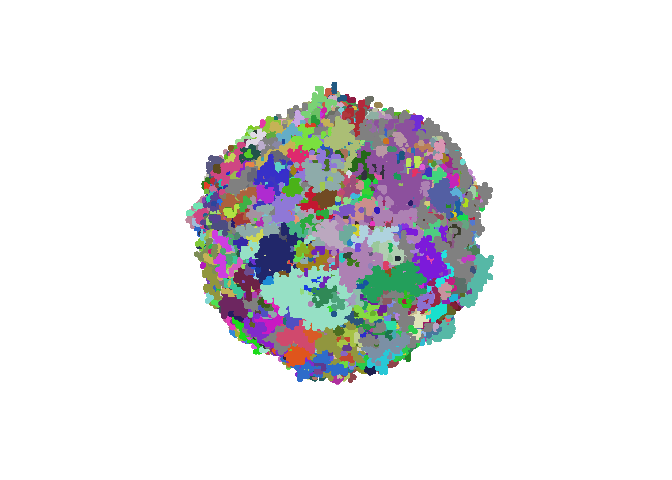Another option is to use plot.type = "heat", which colors cells on a scale from blue to red, depending on the number of mutations within the cell. This allows for the user to observe regions of high mutation counts.

visualizeTumor(out, background = "white", plot.type = "heat")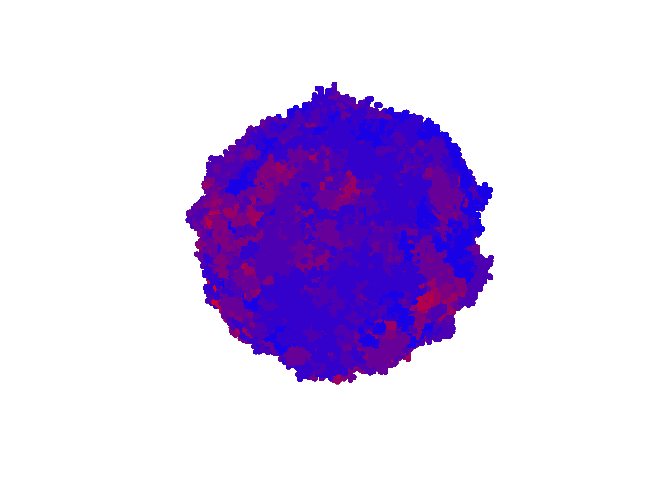One can easily look inside the tumor by plotting a (static) cross-section with the plotSlice() function. One can slice in any coordinate direction and level with the slice.dim and level arguments (see the user manual).

par(mfrow = c(1,2))
plotSlice(tumor = out)
plotSlice(tumor = out, plot.type = "heat")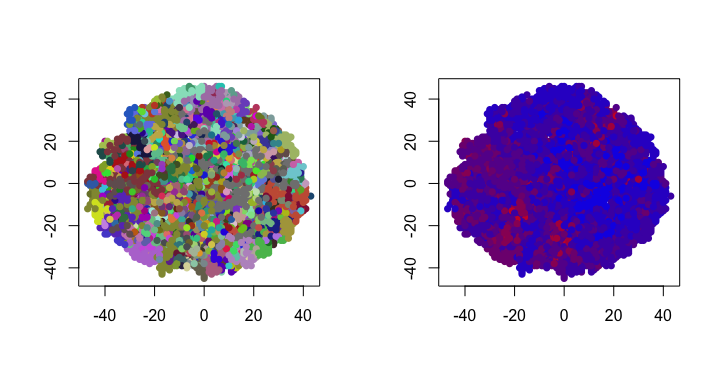## Spatial distribution of mutants

One of the main reasons for using a spatial simulation of tumor growth is to investigate biases in the distribution of mutants throughout the tumor. There are countless questions that can be asked, and hopefully the return value of simulateTumor() will give enough information to answer most of these. To get a quick summary of the spatial distribution of mutations, SITH includes spatialDistribution(). This includes a plot of the number of mutations as a function of Euclidean distance, a histogram of the number of mutations, and the mutations with the largest MAF. We can compare the similarity of two cells $$A$$ and $$B$$ by viewing their genotypes as a binary vector (with component $$i$$ on if mutation $$i$$ is present in the cell) and computing the jaccard index $$J(A,B) = |A \cap B|/|A \cup B|$$. spatialDistribution() also estimates the average Jaccard index of cells as a function of the Euclidean distance between them.

sp <- spatialDistribution(tumor = out)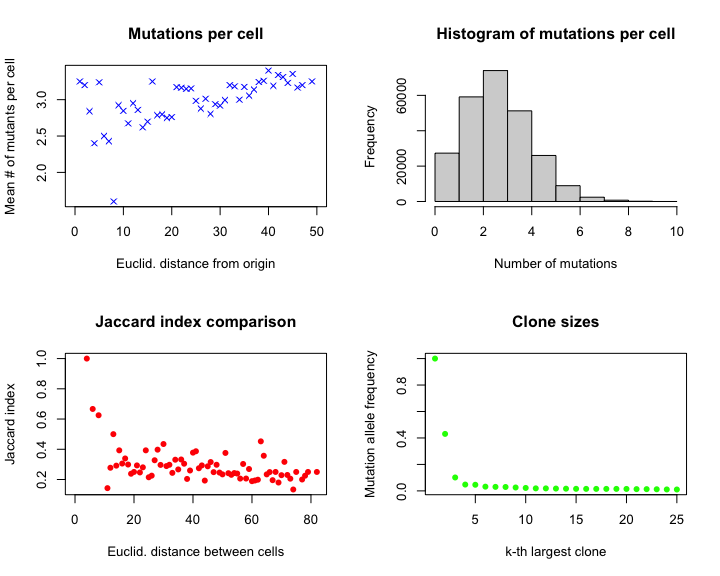Note that the list sp also contains all of the raw data needed to generate the plots.

names(sp)
#>  "mean_mutant" "mean_driver" "jaccard"

## Simulating sequencing data

An exciting application of the spatial models of intratumor heterogeneity is to use them to generate synthetic sequencing data sets.

Recently, there have been a myriad of algorithms designed to infer clonal composition or tumor phylogeny from single cell sequencing or bulk sequencing data sets (for example, Kuipers and Beerenwinkel (2016)). It is not well understood what the impact of spatially biased sampling methods will have on these methods. Using simulated data sets from spatial models could be helpful in determining which inference algorithms are likely to be useful in practice.

SITH contains several functions that simulate single cell sequencing and bulk sampling, allowing the user to get synthetic sequencing data sets from the tumor.

### Single cell sequencing

randomSingleCells() will take random cells from the tumor and report the list of mutations present in each cell. Due to artifacts of sequencing technology, it is expected that there are a large number of false negatives. To account for this, the fnr parameter introduces false negatives into the data set at the specified rate (there is also a fpr parameter for false positives). The function returns a binary matrix, where a $$1$$ indicates that a mutation is present.

Scs <- randomSingleCells(tumor = out, ncells = 5, fnr = 0.1)
head(Scs)
#>      mutID-0 mutID-2 mutID-27304 mutID-72723 mutID-5483 mutID-49311
#> SC-1       1       1           0           0          0           0
#> SC-2       1       0           0           0          0           0
#> SC-3       1       0           1           1          0           0
#> SC-4       0       0           0           0          0           0
#> SC-5       1       1           0           0          1           1

The user can also sequence a single cell at a specified $$(x,y,z)$$ location using the singleCell() function with argument pos = (x,y,z). See the user manual for details.

### Bulk sampling

One can also simulate bulk sampling by taking a collection of cells and calculating the variant allele frequency (VAF) of each mutation. One way to define a collection of cells is to take a $$n \times n \times n$$ cube. Function randomBulkSamples will choose random locations for the cubes. Note that argument cube.length must be odd in order for the cube to have a well-defined center. In practice, mutations that fall a certain VAF ($$\approx 0.05$$) are either ignored or undetected due to technological artifacts. To account for this, the threshold argument åwill ignore mutations below a certain VAF. The user can also specify a desired sequencing depth by adjusting the coverage parameter. If a coverage of $$C$$ is specified, then the number of reads for each base is sampled $$M \sim Pois(C)$$. Then the number of variant calls is sampled from a binomial where the number of trials is $$M$$ and the probability of success is equal to the true VAF of the sample. If you don’t want to simulate deep sequencing then leave coverage blank or set it to $$0$$. The return of the function is a matrix of VAFs.

Bulks <- randomBulkSamples(tumor = out, nsamples = 5, cube.length = 7, coverage=100, threshold = 0.05)
head(Bulks)
#>          mutID-0   mutID-2 mutID-6845 mutID-8724 mutID-19947 mutID-30325   mutID-5  mutID-633 mutID-3339 mutID-414 mutID-5117 mutID-30149 mutID-2217 mutID-5142 mutID-7104 mutID-21006 mutID-22691 mutID-27447 mutID-941 mutID-1840 mutID-28010 mutID-5655 mutID-6297 mutID-17606 mutID-4164 mutID-7355 mutID-738 mutID-187  mutID-597 mutID-5970 mutID-3072 mutID-6096 mutID-2507 mutID-3952 mutID-15681 mutID-17503 mutID-9068
#> Bulk-1 0.7475728 0.6504854  0.0776699  0.1262136  0.08737864  0.02912621 0.1553398 0.08737864 0.06796117 0.1650485 0.02912621  0.06796117  0.1067961  0.0000000  0.0000000   0.0000000   0.0000000  0.00000000 0.0000000  0.0000000  0.00000000  0.0000000  0.0000000   0.0000000  0.0000000  0.0000000 0.0000000 0.0000000 0.00000000  0.0000000  0.0000000 0.00000000  0.0000000  0.0000000    0.000000  0.00000000 0.00000000
#> Bulk-2 0.6504854 0.1262136  0.0000000  0.0000000  0.00000000  0.00000000 0.1165049 0.00000000 0.00000000 0.0000000 0.00000000  0.00000000  0.0000000  0.2135922  0.3009709   0.1650485   0.1165049  0.09708738 0.2427184  0.2427184  0.09708738  0.0000000  0.0000000   0.0000000  0.0000000  0.0000000 0.0000000 0.0000000 0.00000000  0.0000000  0.0000000 0.00000000  0.0000000  0.0000000    0.000000  0.00000000 0.00000000
#> Bulk-3 0.7087379 0.0000000  0.0000000  0.0000000  0.00000000  0.00000000 0.0000000 0.00000000 0.00000000 0.0000000 0.00000000  0.00000000  0.0000000  0.0000000  0.0000000   0.0000000   0.0000000  0.00000000 0.0000000  0.0000000  0.00000000  0.1359223  0.1165049   0.1650485  0.1456311  0.1067961 0.0000000 0.0000000 0.00000000  0.0000000  0.0000000 0.00000000  0.0000000  0.0000000    0.000000  0.00000000 0.00000000
#> Bulk-4 0.8349515 0.3786408  0.0000000  0.0000000  0.00000000  0.00000000 0.4466019 0.00000000 0.00000000 0.0000000 0.00000000  0.00000000  0.0000000  0.0000000  0.0000000   0.0000000   0.0000000  0.00000000 0.0000000  0.0000000  0.00000000  0.0000000  0.0000000   0.0000000  0.0000000  0.0000000 0.2330097 0.1067961 0.05825243  0.0776699  0.0000000 0.00000000  0.0000000  0.0000000    0.000000  0.00000000 0.00000000
#> Bulk-5 0.8446602 0.3689320  0.0000000  0.0000000  0.00000000  0.00000000 0.0000000 0.00000000 0.00000000 0.0000000 0.00000000  0.00000000  0.0000000  0.0000000  0.0000000   0.0000000   0.0000000  0.00000000 0.0000000  0.0000000  0.00000000  0.0000000  0.0000000   0.0000000  0.0000000  0.0000000 0.0000000 0.0000000 0.00000000  0.0000000  0.2427184 0.04854369  0.2135922  0.1747573    0.184466  0.05825243 0.06796117

If instead one would like to choose the location of the cube, bulkSample() can be used and pos can be set to the cube center.

One can also simulate a long, thin needle passing through the tumor to collect a sample, as described in Chkhaidze et. al. (2019). This is implemented as randomNeedles() and takes the same arguments as randomBulkSamples (minus cube.length).

Needs <- randomNeedles(tumor = out, nsamples = 5, threshold = 0.05)
head(Needs)
#>        mutID-0   mutID-2 mutID-183 mutID-24088 mutID-4531 mutID-7388 mutID-47247 mutID-27071 mutID-23708 mutID-56196  mutID-56  mutID-57 mutID-108  mutID-205 mutID-35881 mutID-14003  mutID-331 mutID-33260 mutID-2337  mutID-16 mutID-187 mutID-597 mutID-24362 mutID-16392 mutID-6271 mutID-340 mutID-2548 mutID-2768  mutID-213 mutID-3388 mutID-414 mutID-7302 mutID-7303 mutID-15248 mutID-6075 mutID-2217 mutID-3327  mutID-941 mutID-1975
#> Bulk-1       1 0.4545455 0.1136364   0.1136364  0.1136364 0.06818182  0.09090909        0.00   0.0000000  0.00000000 0.0000000 0.0000000 0.0000000 0.00000000  0.00000000    0.000000 0.00000000  0.00000000 0.00000000 0.0000000 0.0000000 0.0000000  0.00000000   0.0000000 0.00000000 0.0000000 0.00000000 0.00000000 0.00000000 0.00000000 0.0000000 0.00000000 0.00000000  0.00000000        0.0  0.0000000  0.0000000 0.00000000  0.0000000
#> Bulk-2       1 0.0000000 0.0000000   0.0000000  0.0000000 0.00000000  0.00000000        0.25   0.4166667  0.08333333 0.0000000 0.0000000 0.0000000 0.00000000  0.00000000    0.000000 0.00000000  0.00000000 0.00000000 0.0000000 0.0000000 0.0000000  0.00000000   0.0000000 0.00000000 0.0000000 0.00000000 0.00000000 0.00000000 0.00000000 0.0000000 0.00000000 0.00000000  0.00000000        0.0  0.0000000  0.0000000 0.00000000  0.0000000
#> Bulk-3       1 0.9344262 0.0000000   0.0000000  0.0000000 0.00000000  0.00000000        0.00   0.0000000  0.00000000 0.2786885 0.2786885 0.2786885 0.06557377  0.06557377    0.147541 0.06557377  0.08196721 0.06557377 0.1147541 0.0000000 0.0000000  0.00000000   0.0000000 0.00000000 0.0000000 0.00000000 0.00000000 0.00000000 0.00000000 0.0000000 0.00000000 0.00000000  0.00000000        0.0  0.0000000  0.0000000 0.00000000  0.0000000
#> Bulk-4       1 0.4637681 0.0000000   0.0000000  0.1159420 0.11594203  0.00000000        0.00   0.0000000  0.00000000 0.0000000 0.0000000 0.0000000 0.00000000  0.00000000    0.000000 0.00000000  0.00000000 0.00000000 0.0000000 0.2463768 0.2463768  0.08695652   0.1449275 0.05797101 0.1014493 0.07246377 0.07246377 0.05797101 0.00000000 0.0000000 0.00000000 0.00000000  0.00000000        0.0  0.0000000  0.0000000 0.00000000  0.0000000
#> Bulk-5       1 0.9333333 0.0000000   0.0000000  0.0000000 0.00000000  0.00000000        0.00   0.0000000  0.00000000 0.0000000 0.0000000 0.0000000 0.00000000  0.00000000    0.000000 0.00000000  0.00000000 0.00000000 0.0000000 0.0000000 0.0000000  0.00000000   0.0000000 0.00000000 0.0000000 0.00000000 0.00000000 0.00000000 0.08888889 0.1333333 0.08888889 0.08888889  0.08888889        0.2  0.1555556  0.1555556 0.06666667  0.1111111

There is currently no function allowing the user to select the location of the user, although this could be included in future versions.

## User-defined mutations

By default, simulateTumor() assumes the “infinite alleles hypothesis.” This means that each mutation only occurs once (so that every new mutation is unique). While this is useful for investigating how genetic diversity is organized within an individual tumor, it doesn’t allow the user any control over what mutations can occur and at what rate. For example, the user may want to simulate a population of tumors according to an underlying disease model.

### Defining a disease model

Suppose that there are $$n$$ genetic mutations of interests. A fully expressive model would need to define $$2^n$$ birth rates corresponding to each possible subsets of mutations that can be present in a cell’s genome. While this is possible for small $$n$$, it quickly becomes intractable. For this reason, we choose a more restricting parameterization.

Our model assumes that there is a directed acyclic graph (DAG) $$G$$ governing the order in which mutations can occur. The vertices of $$G$$ are the mutations and the edges constrain the order in which the mutations occur. In particular, suppose that a cell’s genotype contains mutations $$V' \subset V$$. During division, we assume that a cell can acquire any mutation $$m$$ for which there is an edge $$(v',m) \in E$$ such that $$v' \in V'$$. Furthermore, we assume that each edge $$e = (v,w)$$ has a mutation rate $$u_e$$ which gives the probability that a cell with mutation $$v$$ acquires mutation $$w$$ during division. When crossing edge $$e$$, the birth rate of the cell multiplied by $$s_e$$ (the selective advantage).

To summarize the above paragraph, we require a DAG $$G$$, probabilities for cross each edge $$u_e$$, and selective advantages for crossing each edge $$s_e$$.

The simplest way to encode the above information is with a matrix with $$|E|$$ rows and $$4$$ columns. Function progressionChain() generates a simple example where $$G$$ is a linear chain with $$n$$ vertices:

library(SITH)
set.seed(278115205)

n <- 5
G <- progressionChain(5)
G
#>      Head Tail Mut. rate Selective advantage
#> [1,]    0    1     0.001                   1
#> [2,]    1    2     0.001                   1
#> [3,]    2    3     0.001                   1
#> [4,]    3    4     0.001                   1
#> [5,]    4    5     0.001                   1

The matrix returned by progressionChain() is the input that is required to simulateTumor(). Of course, the user can change head and tail to create more complicated DAGs.

Once we have obtained a matrix of the desired form, we can plug it right into the disease model parameter simulateTumor().

G[,3] <- rep(0.02, nrow(G))
out <- simulateTumor(max_pop = 1000, disease_model=G)
#> Initializing structures ... ...
#> Simulation complete. Releasing memory ... ...
#> Simulated time is 83.5229 days
#> Simulation completed in 0.047088 s.
#> Writing results ... ...

The out list is exactly the same as the returned by simulateTumor(). In particular all of the downstream functions described in the first vignette apply to the output of simulateTumorUDT().

We defined the disease model G to be a linear chain with $$5$$ vertices. We should thus expect mutation $$0$$ to be less frequent than mutation $$1$$, since $$0$$ is required for $$1$$ to occur.

out$muts #> id count MAF #> 1 0 1000 1.000 #> 2 1 323 0.323 #> 3 2 12 0.012 Let’s also take a look at a 2D cross section plotSlice(out)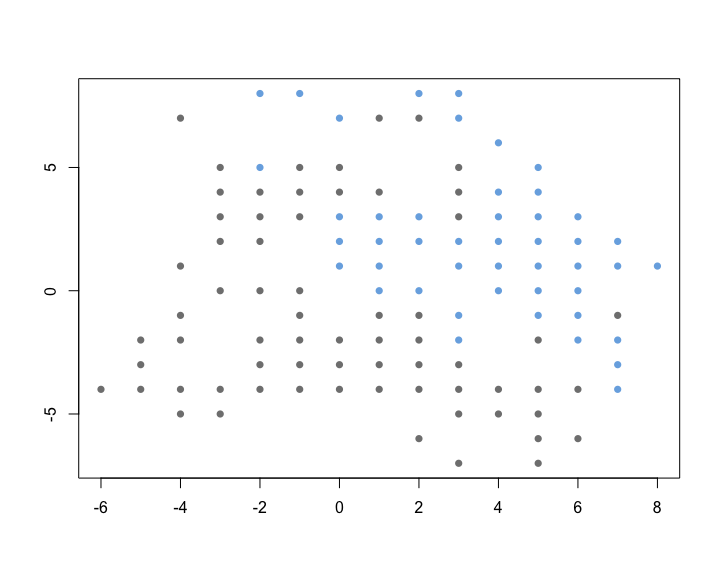### Converting from igraph To define complicated networks, it is probably easier to obtain the matrix G from an igraph object. We provide functionality to do this. Let’s start by whipping up a simple DAG: if (!requireNamespace("igraph", quietly = TRUE)) { stop("igraph needed to compile the vignette.", call. = FALSE) } el <- matrix(as.character(c(0,1,0,2,2,3,3,4,2,5,1,5)), ncol = 2, byrow = TRUE) iG <- igraph::graph_from_edgelist(el) plot(iG)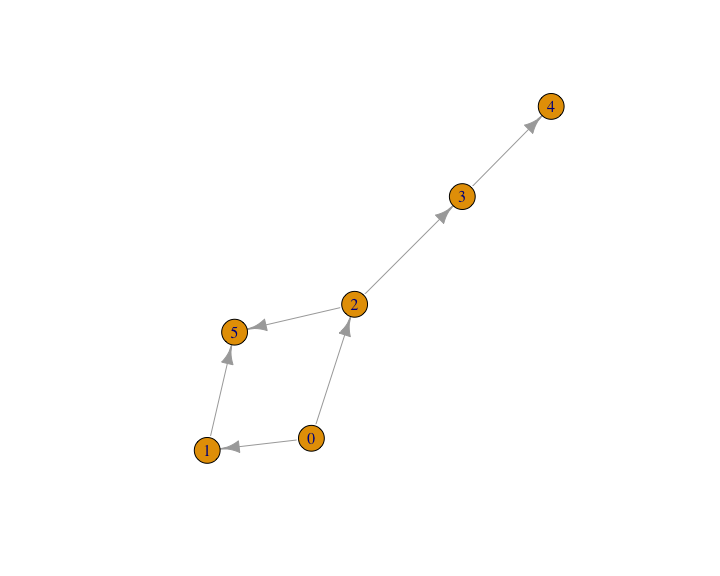We’ll assume that $$0$$ is the initial mutation (similar to the original model). Now we can convert this object into a matrix that can be used by simulateTumor() using progressionDAG_from_igraph(). G <- progressionDAG_from_igraph(iG) G #> Head Tail Mut. rate Selective advantage #> [1,] 0 1 0.001 1 #> [2,] 0 2 0.001 1 #> [3,] 2 3 0.001 1 #> [4,] 3 4 0.001 1 #> [5,] 2 5 0.001 1 #> [6,] 1 5 0.001 1 And now we can simulate a tumor according to these constraints: out <- simulateTumor(max_pop=5000,disease_model=G, verbose = FALSE) out$genotypes
#>   X1 X2 X3 count
#> 1  0 -1 -1  4744
#> 2  0  1 -1   176
#> 3  0  2 -1    73
#> 4  0  1  2     7
#> 5  0  1  5     0

### Modeling loss-of-function mutations

A loss-of-function mutation usually requires both copies of the gene to be mutated. A tumor cell may gain a selective advantage if a loss-of-function mutation occurs in the tumor suppressor gene TP53. However, it is possible that the cell is at a disadvantage if only one of the two copies are mutated. This might be referred to as “crossing the fitness valley.”

simulateTumor() is well-suited for studying a model of loss-of-function mutations.

We can model mutations in TP53 as follows: state $$0$$ corresponds to the wild-type allele, state $$1$$ corresponds to a cell with $$1$$ copy of TP53, and cell $$2$$ corresponds to a cell with both copies of TP53 mutated. Furthermore, we will assume that a cell with only $$1$$ mutated copy of TP53 has a selective disadvantage. However, a cell with mutations in both copies of TP53 has a selective advantage. For simplicity, let’s assume that both copies can’t be lost in a single event, so that state $$2$$ cells can only arise from state $$1$$ cells. Using the functionality described above, we can simulate this model in just a few lines of code.

# Make a chain: 0 -> 1 -> 2
G <- progressionChain(2)

#Give a disadvantage to a cell in state 1 and an advantage to a cell in state 2
G[1,4] <- 0.9; G[2,4] <- 1.4
G[,3] <- c(0.005,0.005)
G
#>      Head Tail Mut. rate Selective advantage
#> [1,]    0    1     0.005                 0.9
#> [2,]    1    2     0.005                 1.4

#Simulate the model with N = 250000 cells
out <- simulateTumor(disease_model=G)
#> Initializing structures ... ...
#> Simulated time: 301.088 days. Population is 86602 cells.
#> Simulated time: 335.081 days. Population is 157408 cells.
#> Simulated time: 356.48 days. Population is 223625 cells.
#> Simulation complete. Releasing memory ... ...
#> Simulated time is 363.48 days
#> Simulation completed in 2.40094 s.
#> Writing results ... ...

Let’s see what a cross section looks like:

plotSlice(out)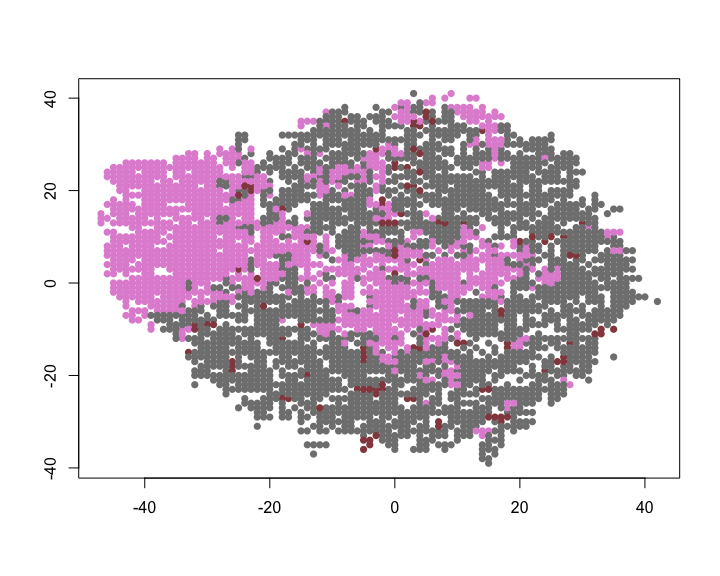Cells with one copy of the gene mutated appear to spread out somewhat evenly while cells with both copies mutated are clustered together. Remember, if you are ever confused about which color is which just check:

out$color_scheme #>  "#808080FF" "#934A4EFF" "#E191D5FF" Let’s also check the MAFs out$muts
#>   id  count      MAF
#> 1  0 250000 1.000000
#> 2  1 166007 0.664028
#> 3  2 161213 0.644852

out\$genotypes
#>   X1 X2 X3  count
#> 1  0 -1 -1  83993
#> 2  0  1 -1   4794
#> 3  0  1  2 161213

Of course, all of the other functions that we used in the introduction vignette can be applied to analyze this model as well.

## Appendix

### The simulation algorithm

The model is simulated using a Gillespie algorithm (Gillespie 1977). Given a population of $$N$$ cells at time $$t$$, cell $$i$$ is chosen to replicate with probability $$b_i/\sum_{j=1}^N (b_j + d_j)$$ (provided a free space is available) and die with probability $$d_i/\sum_{j=1}^N(b_j + d_j)$$. After an event is selected, the time is updated to be $$t + X$$, where $X \sim Expo \left( \sum_{j=1}^N b_j + d_j \right)$ Our simulation algorithm approximates the parameter of the exponential distribution with $$p_{max} = N \max_j (b_j + d_j)$$.

A standard approach is to use inverse transform sampling to select a cell for replication or death. However, this requires computing a cumulative sum over the birth and death rates for all unique alleles in the population, which is likely to scale linearly with $$N$$. We circumvent this issue by using rejection sampling. On each iteration, a random cell $$i$$ is selected uniformly from the population. Next, we obtain a sample $$u$$ from the distribution over $$[0, p_{max}]$$, where $$p_{max}$$ is as above. If $$u < b_i + d_i$$, then cell $$i$$ is selected to replicate or die. Otherwise, we proceed to the next iteration. Although there are contrived examples where rejection sampling is inefficient, the expected run-time is nearly constant for reasonable parameter values.

### Session information

sessionInfo()
#> R version 4.0.2 (2020-06-22)
#> Platform: x86_64-apple-darwin17.0 (64-bit)
#> Running under: macOS Catalina 10.15.7
#>
#> Matrix products: default
#> BLAS:   /Library/Frameworks/R.framework/Versions/4.0/Resources/lib/libRblas.dylib
#> LAPACK: /Library/Frameworks/R.framework/Versions/4.0/Resources/lib/libRlapack.dylib
#>
#> locale:
#>  C/en_US.UTF-8/en_US.UTF-8/C/en_US.UTF-8/en_US.UTF-8
#>
#> attached base packages:
#>  stats     graphics  grDevices utils     datasets  methods   base
#>
#> other attached packages:
#>  SITH_1.1.0
#>
#> loaded via a namespace (and not attached):
#>   igraph_1.2.5            Rcpp_1.0.5              knitr_1.30              magrittr_1.5            scatterplot3d_0.3-41    xtable_1.8-4            R6_2.4.1                rlang_0.4.7             fastmap_1.0.1           stringr_1.4.0           tools_4.0.2             webshot_0.5.2           xfun_0.17               miniUI_0.1.1.1          htmltools_0.5.0         crosstalk_1.1.0.1       yaml_2.2.1              digest_0.6.25           rgl_0.100.54            shiny_1.5.0             later_1.1.0.1           htmlwidgets_1.5.1       promises_1.1.1          manipulateWidget_0.10.1 evaluate_0.14           mime_0.9                rmarkdown_2.5           stringi_1.5.3           compiler_4.0.2          jsonlite_1.7.1          httpuv_1.5.4            pkgconfig_2.0.3